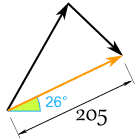# 矢量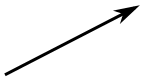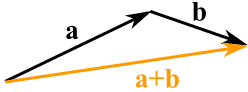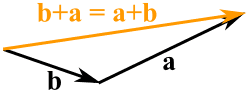### 例子：飞机向北飞，风从西北吹来。## 减

• 先把要减去的矢量倒转方向，
• 然后相加：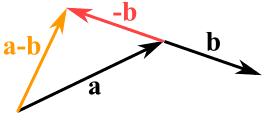ab

## 记法

 矢量也可以写成 它的头（始点）和尾（终点）的字母，上面再放个箭头，像这样：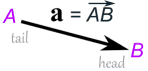## 运算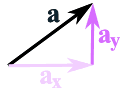（我们会在 下面 解释。）

## 加矢量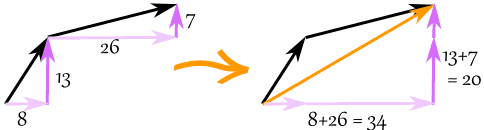c = a + b

c = (8,13) + (26,7) = (8+26,13+7) = (34,20)

## 减矢量

a = v + −k

a = (12,2) + −(4,5) = (12,2) + (−4,−5) = (12−4,2−5) = (8,−3)

## 矢量的量值

|a|

||a||

|a| = √( x2 + y2 )

|b| = √( 62 + 82 ) = √( 36+64 ) = √100 = 10

## 矢量与标量

• 所以 c 是矢量，有量值和方向
• 但 c 只一个值，像 3 或 12.4

## 矢量乘以标量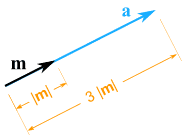a = 3m = (3×7,3×3) = (21,9)

## 矢量乘以矢量（点积和叉积）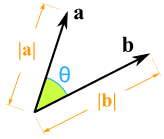怎样把两个矢量相乘？有两一个方法！ 标积（也称点积） （结果是标量）。 矢积（也称叉积） （结果是标量）。 （阅读这些网页来了解更多。）

## 多过 2维### 例子：把矢量 a = (3,7,4) 和 b = (2,9,11) 相加

c = a + b

c = (3,7,4) + (2,9,11) = (3+2,7+9,4+11) = (5,16,15)

### 例子：矢量 w = (1,−2,3) 的量值是多少？

|w| = √( 12 + (−2)2 + 32 ) = √( 1+4+9 ) = √14

### 例子：把 (1,2,3,4) 从 (3,3,3,3) 减去

(3,3,3,3) + −(1,2,3,4)
= (3,3,3,3) + (−1,−2,−3,−4)
= (3−1,3−2,3−3,3−4)
= (2,1,0,−1)

## 量值与方向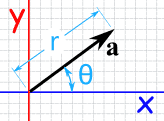<=>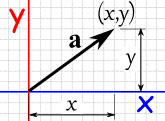极坐标里的 矢量 a 迪卡儿坐标里的矢量 a

极坐标 (r,θ) 迪卡儿坐标 (x,y) 转换为 迪卡儿坐标 (x,y) 转换为 极坐标 (r,θ) x = r × cos( θ ) y = r × sin( θ ) r = √ ( x2 + y2 ) θ = tan-1 ( y / x )## 例子

• 小山 以 200牛顿 的力向 60°的方向拉
• 小王 以 120牛顿 的力向 45°的方向拉 （如图）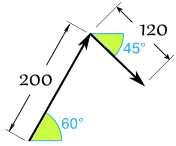• x = r × cos( θ ) = 200 × cos(60°) = 200 × 0.5 = 100
• y = r × sin( θ ) = 200 × sin(60°) = 200 × 0.8660 = 173.21

• x = r × cos( θ ) = 120 × cos(-45°) = 120 × 0.7071 = 84.85
• y = r × sin( θ ) = 120 × sin(-45°) = 120 × -0.7071 = −84.85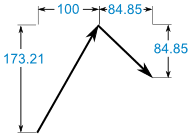(100, 173.21) + (84.85, −84.85) = (184.85, 88.36)

• r = √ ( x2 + y2 ) = √ ( 184.852 + 88.362 ) = 204.88
• θ = tan-1 ( y / x ) = tan-1 ( 88.36 / 184.85 ) = 25.5°